# SAT II Math II : Solving Quadratic Functions

## Example Questions

### Example Question #9 : Solving Functions

A baseball is thrown straight up with an initial speed of 60 feet per second by a man standing on the roof of a 100-foot high building. The height of the baseball in feet as a function of timein seconds is modeled by the function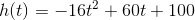To the nearest tenth of a second, how long does it take for the baseball to hit the ground?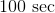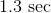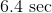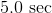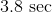Explanation:

When the baseball hits the ground, the height is 0, so we set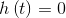. and solve for.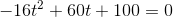This can be done using the quadratic formula: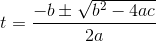Set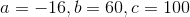: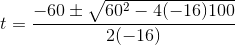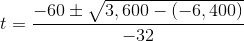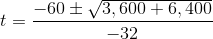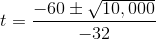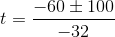One possible solution: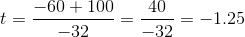We throw this out, since time must be positive.

The other: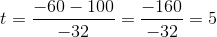This solution, we keep. The baseball hits the ground in 5 seconds.

### All SAT II Math II Resources